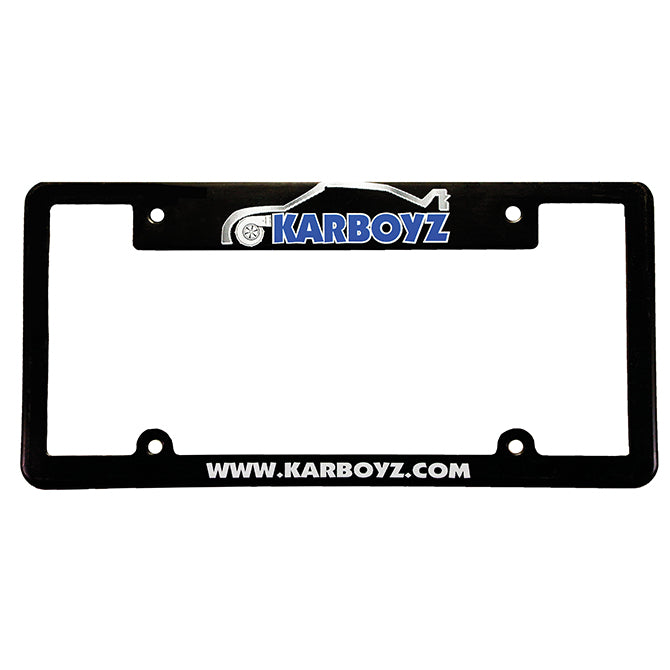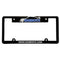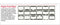Screen printed frames

• Polystyrene frame available in black or white
• Available in frame styles: A, B, D, E, G, H, J, L, R

Pricing:

• 250= \$1.30 each
• 500= .93 each
• 1000= .82 each
• 2500= .77 each
• 5000= .73 each
• 250= .32 each
• 500= .24 each
• 1000= .17 each
• 2500= .14 each
• 5000= .12 each
Vertical text:
• 250= .30 each
• 500= .22 each
• 1000= .15 each
• 2500= .12 each
• 5000= .10 each

*3-4 week production time

*\$75 setup charge

*All orders subject to +/- 5% overrun charge.